## 1. 什么是激活函数

• 激活函数是人工神经网络的一个极其重要的特征；
• 激活函数决定一个神经元是否应该被激活，激活代表神经元接收的信息与给定的信息有关；
• 激活函数对输入信息进行非线性变换，然后将变换后的输出信息作为输入信息传给下一层神经元。

## 3. 激活函数的种类

### 3.1. identity

$$f(x)=x$$

$${ f }^{ ' }(x)=1$$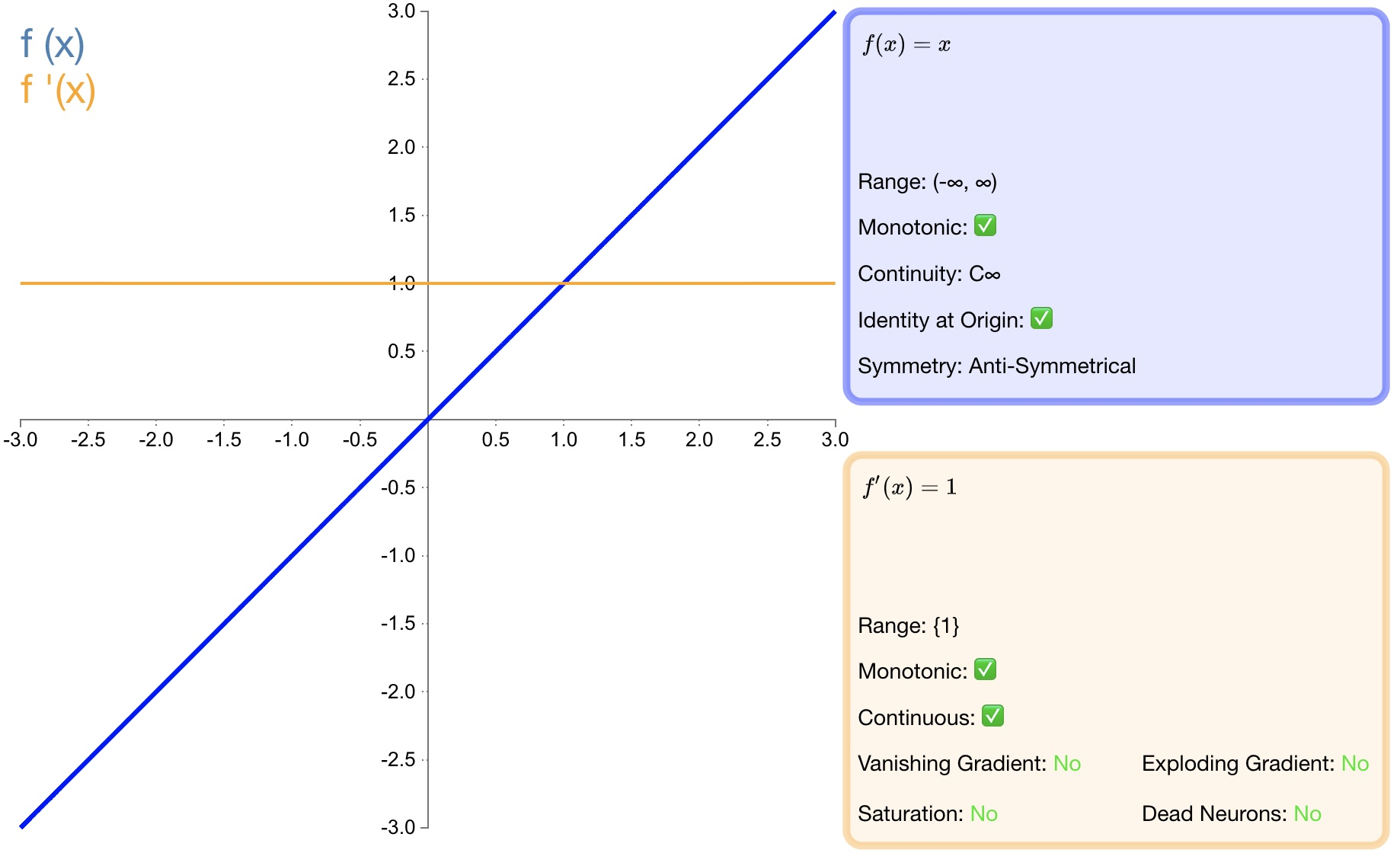### 3.2. step

$${ f }(x)=\begin{cases} \begin{matrix} 0 & x<0 \end{matrix} \\ \begin{matrix} 1 & x\ge 0 \end{matrix} \end{cases}$$

$${ f }^{ ' }(x)=\begin{cases} \begin{matrix} 0 & x\neq 0 \end{matrix} \\ \begin{matrix} ? & x=0 \end{matrix} \end{cases}$$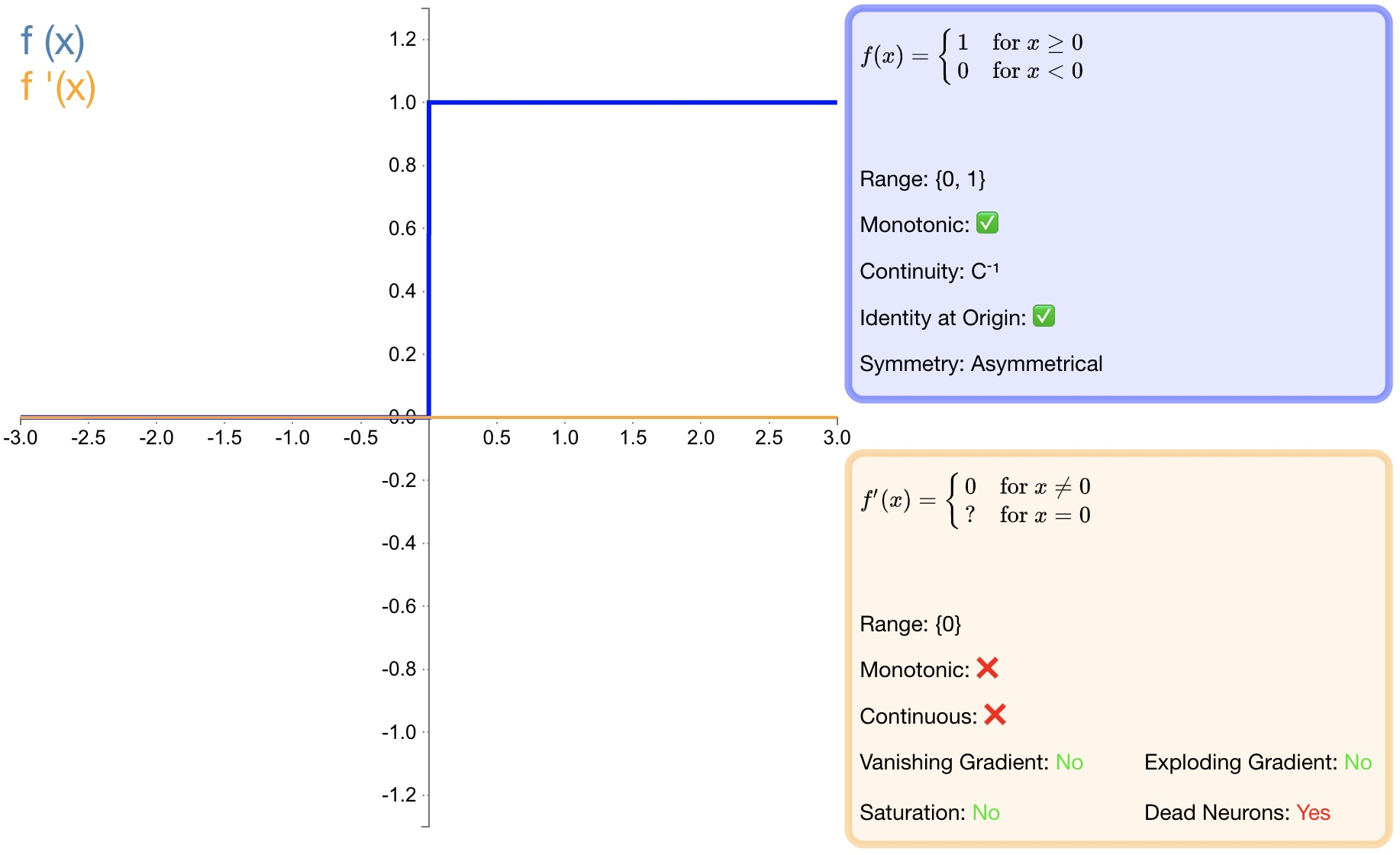### 3.3. sigmoid

$${ f }(x)=\sigma (x)=\frac { 1 }{ 1+{ e }^{ -x } }$$

$${ f }^{ ' }(x)=f(x)(1-f(x))$$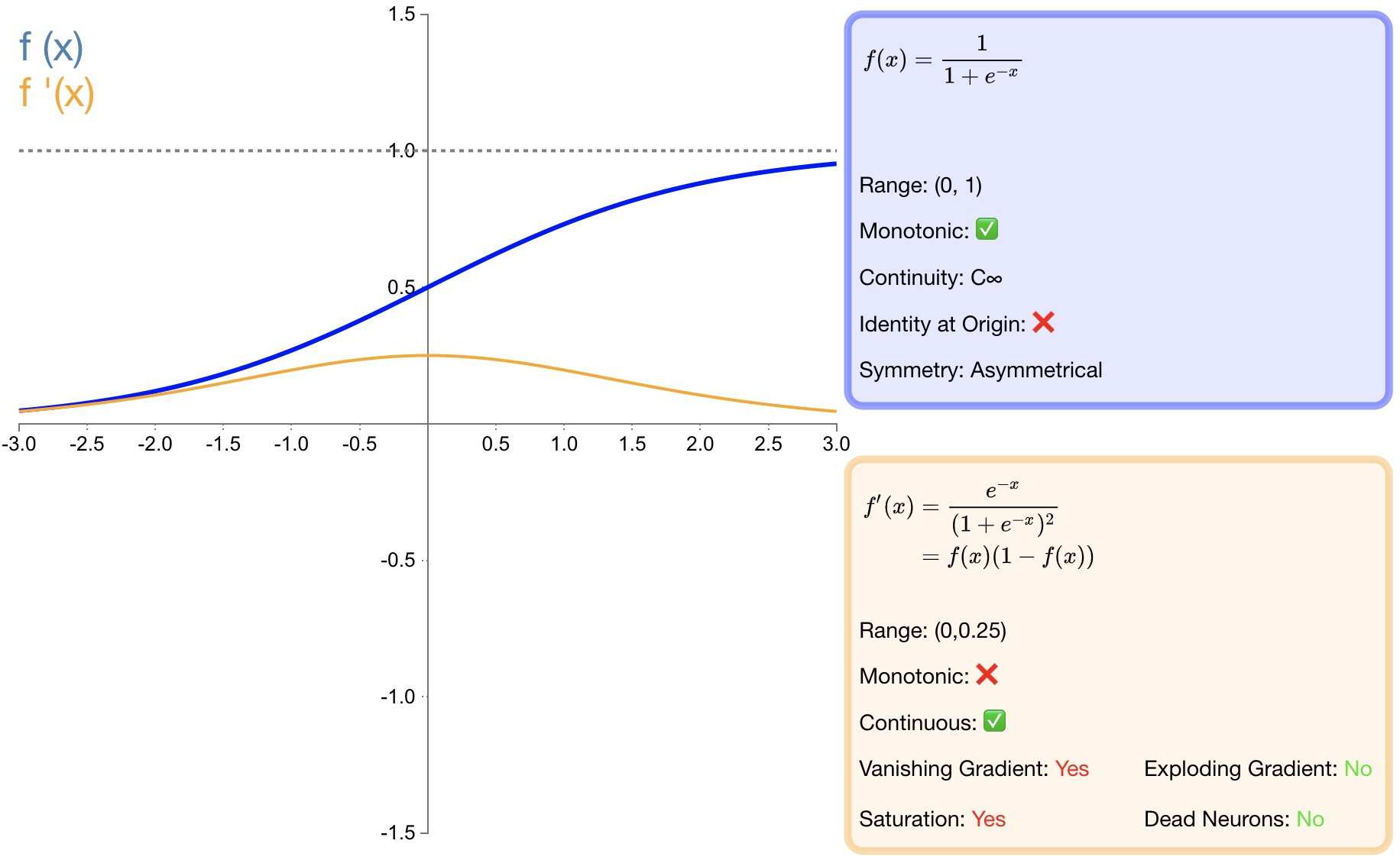- $sigmoid$ 函数的输出映射在 $(0,1)$ 之间，单调连续，输出范围有限，优化稳定，可以用作输出层；

 - 求导容易；

 - 由于其软饱和性，一旦落入饱和区梯度就会接近于0，根据反向传播的链式法则，容易产生梯度消失，导致训练出现问题；

 - 其输出并不是以 $0$ 为中心的，假设输入均为正数（或负数），那么对w的导数总是正数（或负数），这样在反向传播过程中要么都往正方向更新，要么都往负方向更新，导致有一种捆绑效果，使得收敛缓慢；

 - 计算时，由于具有幂运算，计算复杂度较高，运算速度较慢。

### 3.4. tanh

$${ f }(x)=tanh(x)=\frac { { e }^{ x }-{ e }^{ -x } }{ { e }^{ x }+{ e }^{ -x } }$$

$${ f }^{ ' }(x)=1-f(x)^{ 2 }$$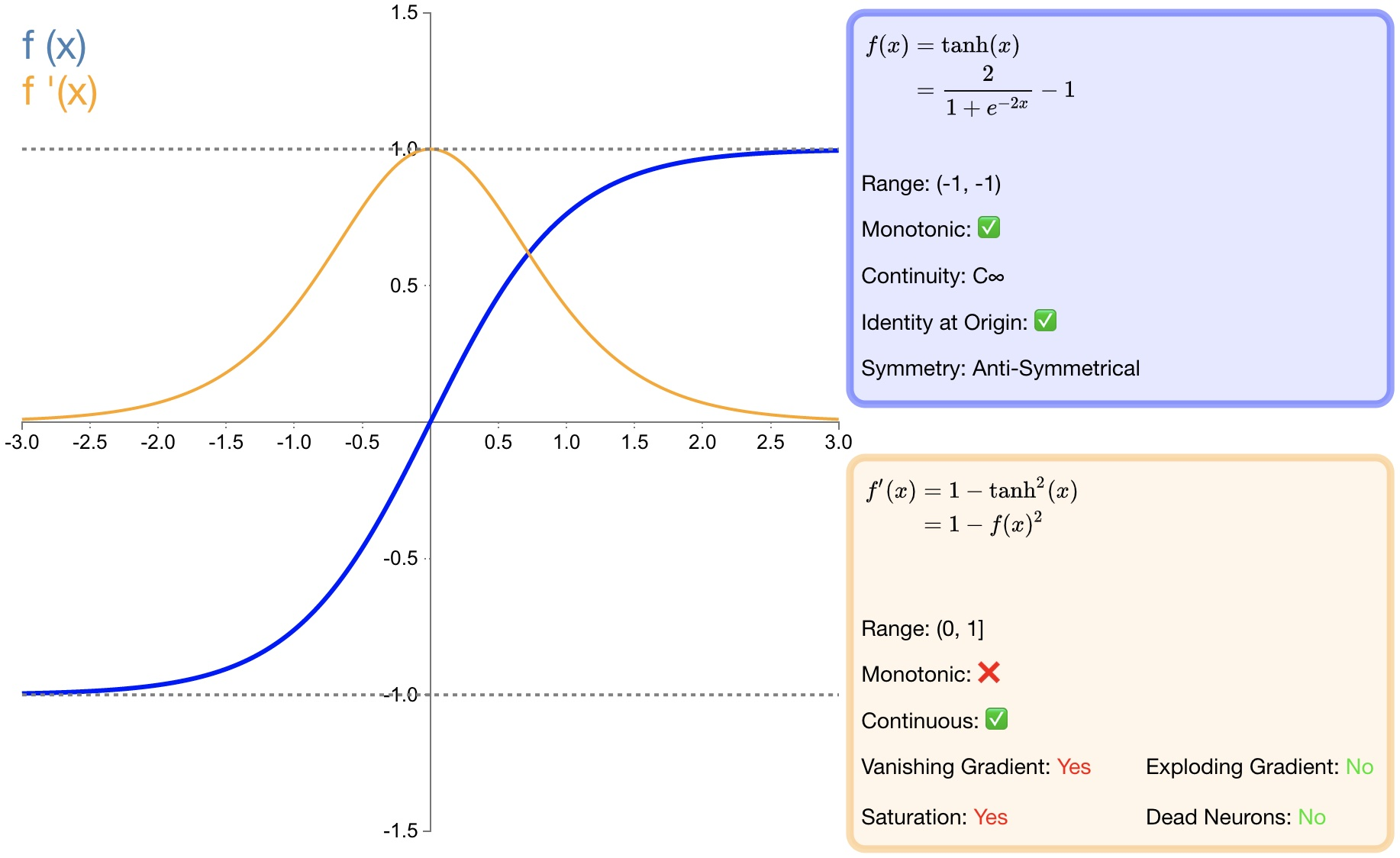- $tanh$ 比 $sigmoid$ 函数收敛速度更快；

 - 相比 $sigmoid$ 函数，$tanh$ 是以 $0$ 为中心的；

 - 与 $sigmoid$ 函数相同，由于饱和性容易产生的梯度消失；

 - 与 $sigmoid$ 函数相同，由于具有幂运算，计算复杂度较高，运算速度较慢。

### 3.5. ReLU

$$f(x)=\begin{cases} \begin{matrix} 0 & x<0 \end{matrix} \\ \begin{matrix} x & x\ge 0 \end{matrix} \end{cases}$$

$${ { f }(x) }^{ ' }=\begin{cases} \begin{matrix} 0 & x<0 \end{matrix} \\ \begin{matrix} 1 & x\ge 0 \end{matrix} \end{cases}$$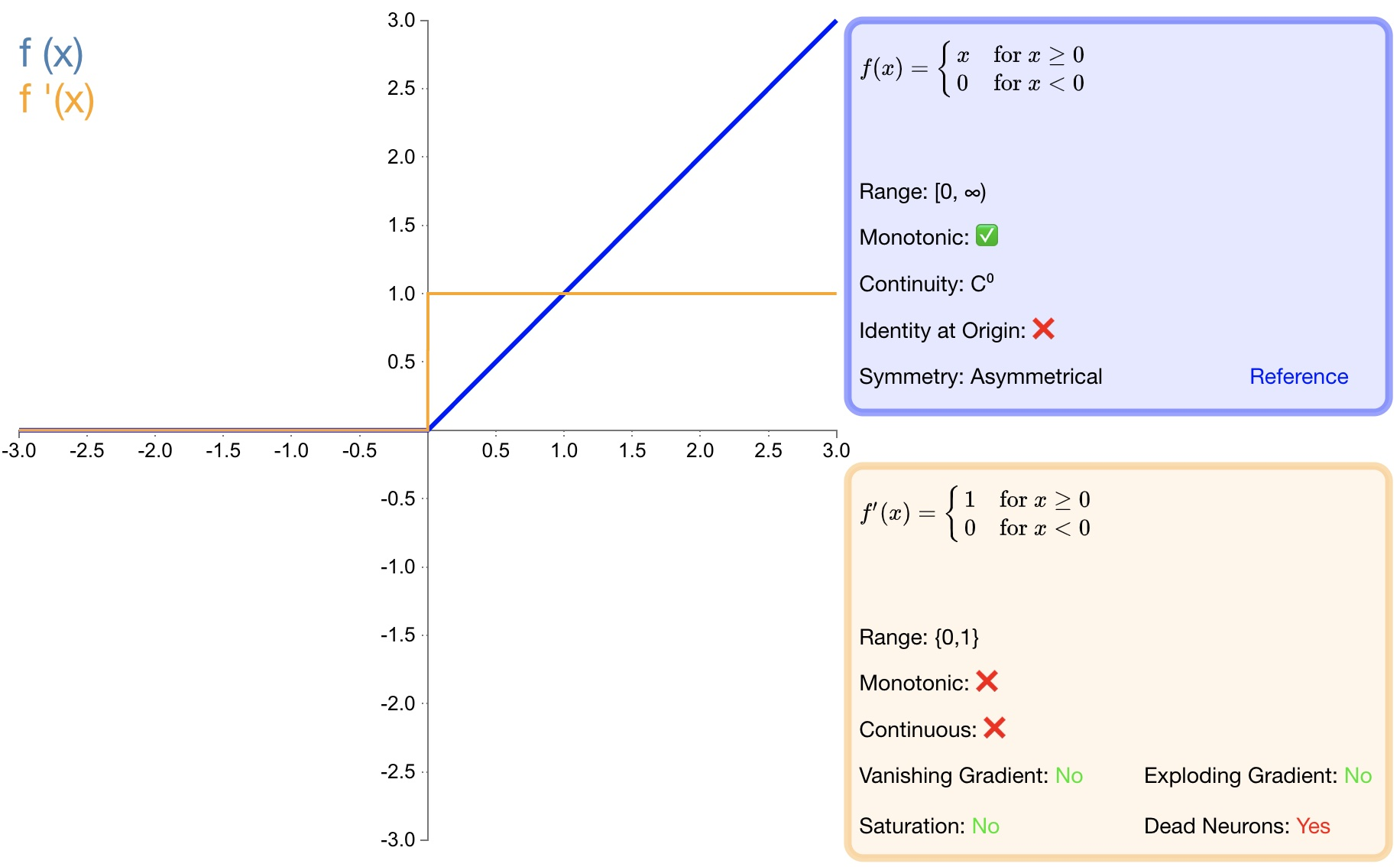- 收敛速度快；

 - 相较于 $sigmoid$ 和 $tanh$ 中涉及了幂运算，导致计算复杂度高， ReLU可以更加简单的实现；

 - 当输入 $x>=0$ 时，ReLU 的导数为常数，这样可有效缓解梯度消失问题；

 - 当 $x<0$ 时，ReLU 的梯度总是 $0$，提供了神经网络的稀疏表达能力；

 - ReLU 的输出不是以 $0$ 为中心的；

 - 神经元坏死现象，某些神经元可能永远不会被激活，导致相应参数永远不会被更新；

 - 不能避免梯度爆炸问题；

### 3.6. LReLU

$$f(x)=\begin{cases} \begin{matrix} \alpha x & x<0 \end{matrix} \\ \begin{matrix} x & x\ge 0 \end{matrix} \end{cases}$$

$${ { f }(x) }^{ ' }=\begin{cases} \begin{matrix} \alpha & x<0 \end{matrix} \\ \begin{matrix} 1 & x\ge 0 \end{matrix} \end{cases}$$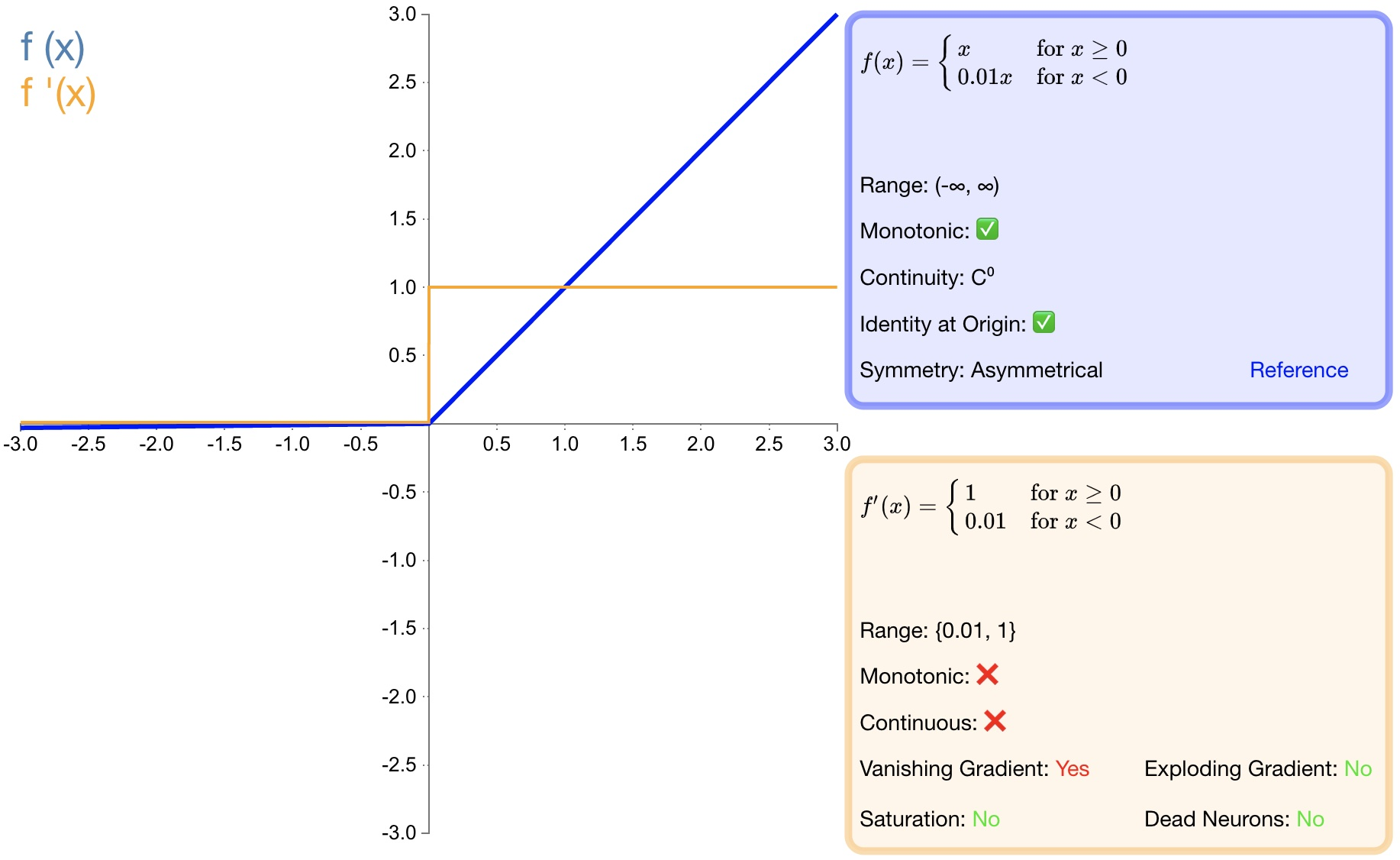- 避免梯度消失；

 - 由于导数总是不为零，因此可减少死神经元的出现；

 - LReLU 表现并不一定比 ReLU 好；

 - 无法避免梯度爆炸问题；

### 3.7. PReLU

$$f(\alpha ,x)=\begin{cases} \begin{matrix} \alpha x & x<0 \end{matrix} \\ \begin{matrix} x & x\ge 0 \end{matrix} \end{cases}$$

$${ { f }(\alpha ,x) }^{ ' }=\begin{cases} \begin{matrix} \alpha & x<0 \end{matrix} \\ \begin{matrix} 1 & x\ge 0 \end{matrix} \end{cases}$$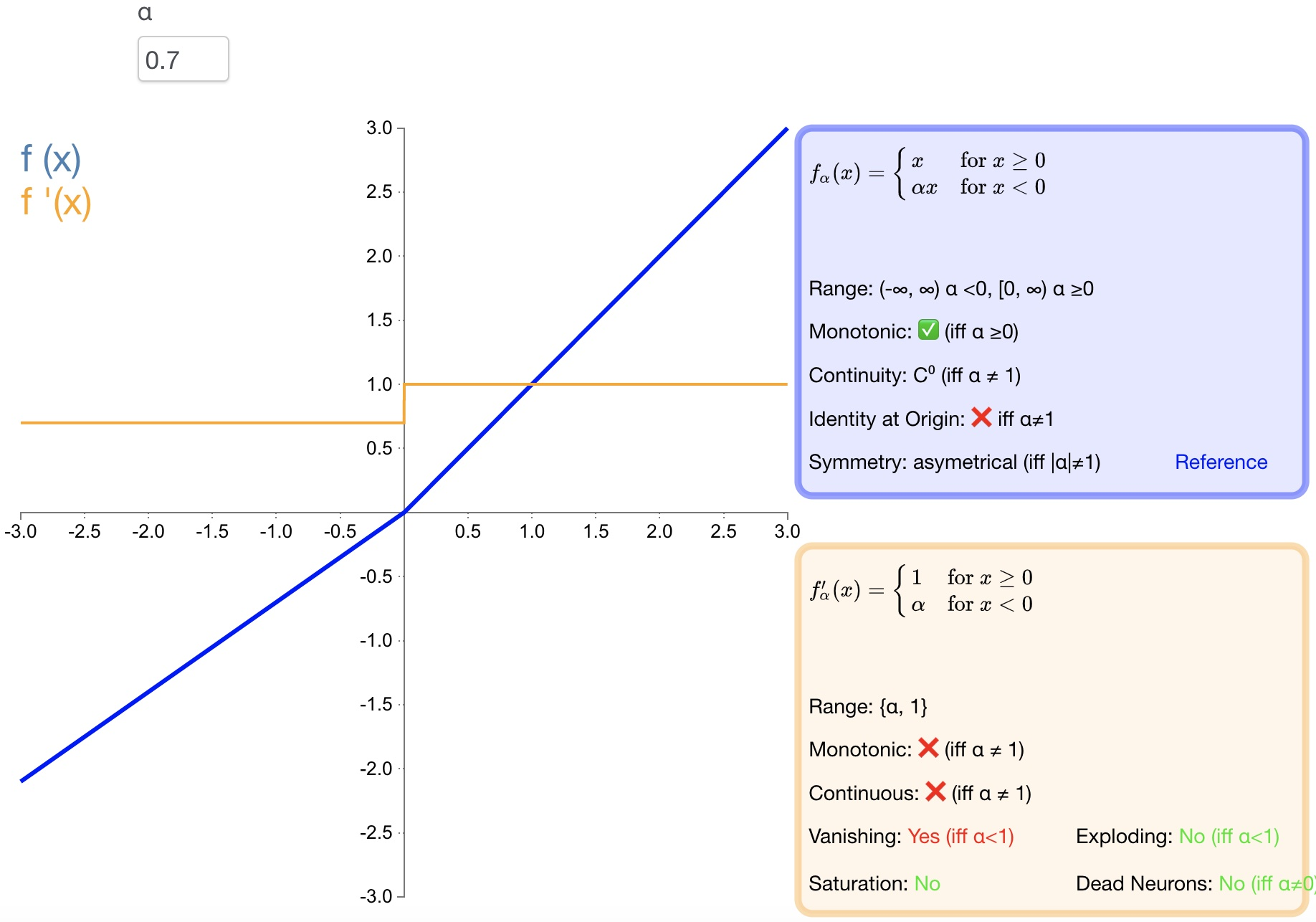- PReLU 是 LReLU 的改进，可以自适应地从数据中学习参数；

 - 收敛速度快、错误率低；

 - PReLU 可以用于反向传播的训练，可以与其他层同时优化；

### 3.8. RReLU

$$f(\alpha ,x)=\begin{cases} \begin{matrix} \alpha x & x<0 \end{matrix} \\ \begin{matrix} x & x\ge 0 \end{matrix} \end{cases}$$

$${ { f }(\alpha ,x) }^{ ' }=\begin{cases} \begin{matrix} \alpha & x<0 \end{matrix} \\ \begin{matrix} 1 & x\ge 0 \end{matrix} \end{cases}$$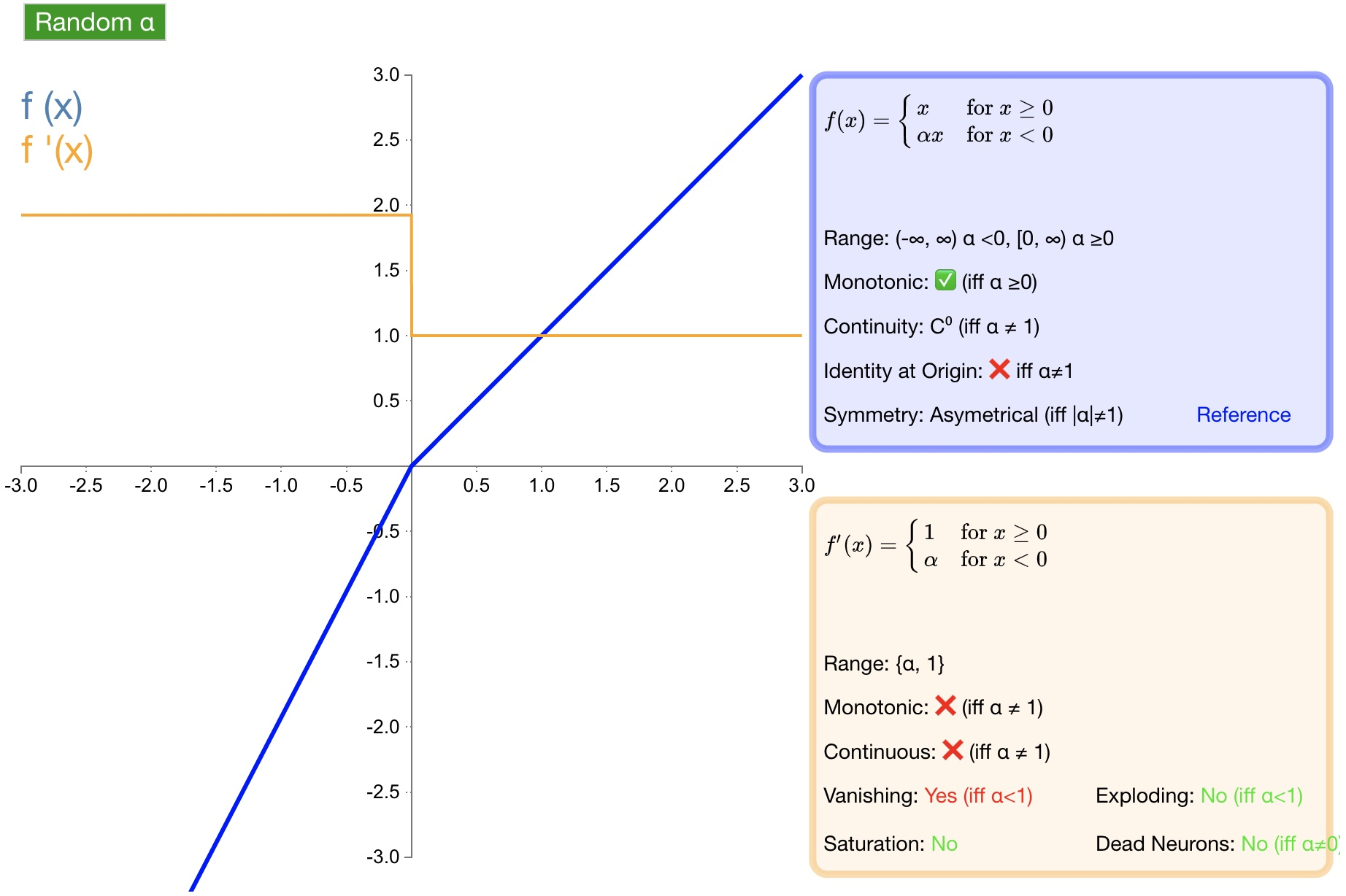### 3.9. ELU

$$f(\alpha ,x)=\begin{cases} \begin{matrix} \alpha \left( { e }^{ x }-1 \right) & x<0 \end{matrix} \\ \begin{matrix} x & x\ge 0 \end{matrix} \end{cases}$$

$${ { f }(\alpha ,x) }^{ ' }=\begin{cases} \begin{matrix} f(\alpha ,x)+\alpha & x<0 \end{matrix} \\ \begin{matrix} 1 & x\ge 0 \end{matrix} \end{cases}$$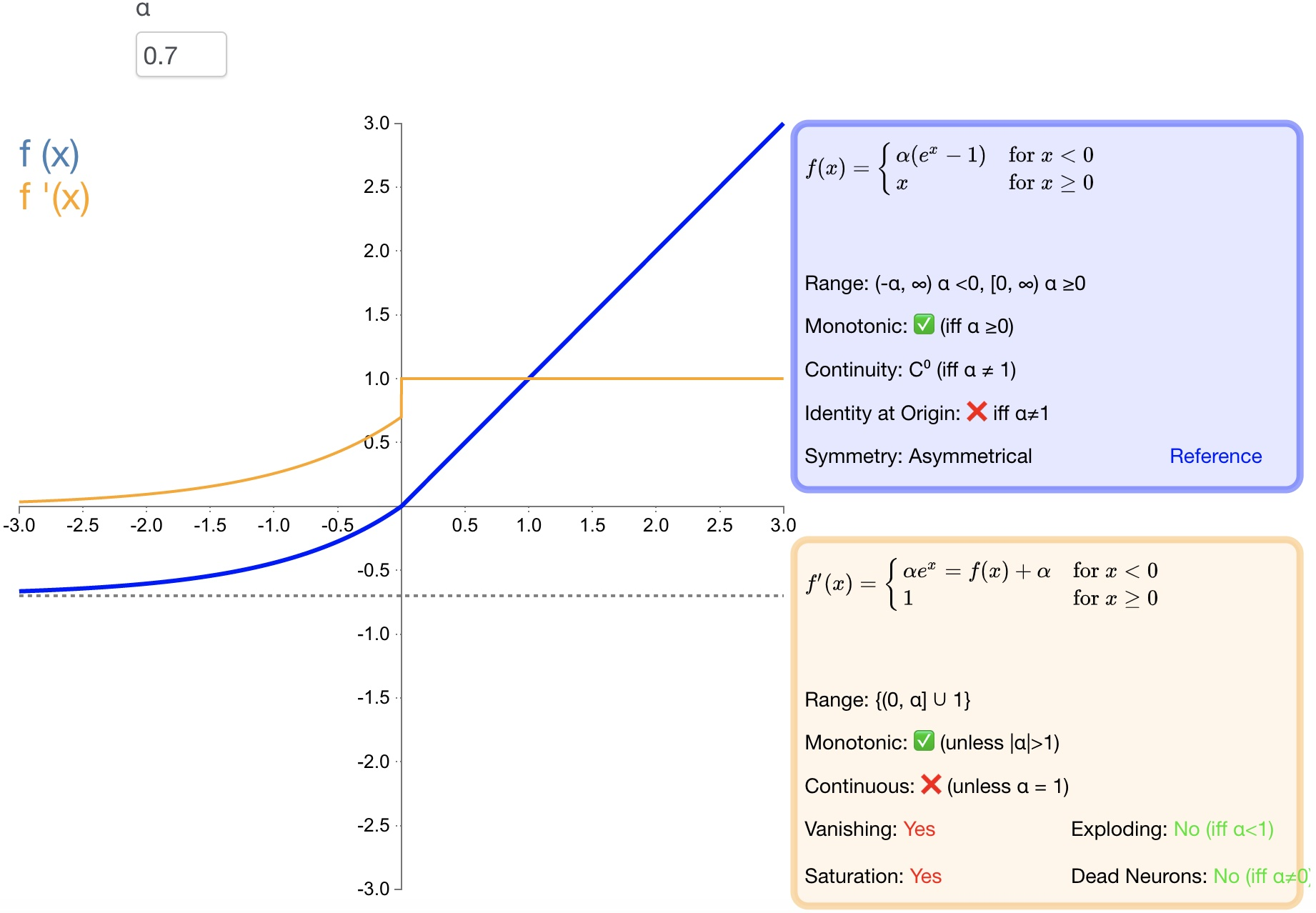- 导数收敛为零，从而提高学习效率；

 - 能得到负值输出，这能帮助网络向正确的方向推动权重和偏置变化；

 - 防止死神经元出现。

 - 计算量大，其表现并不一定比 ReLU 好；

 - 无法避免梯度爆炸问题；

### 3.10. SELU

$$f(\alpha ,x)=\lambda \begin{cases} \begin{matrix} \alpha \left( { e }^{ x }-1 \right) & x<0 \end{matrix} \\ \begin{matrix} x & x\ge 0 \end{matrix} \end{cases}$$

$${ { f }(\alpha ,x) }^{ ' }=\lambda \begin{cases} \begin{matrix} \alpha \left( { e }^{ x } \right) & x<0 \end{matrix} \\ \begin{matrix} 1 & x\ge 0 \end{matrix} \end{cases}$$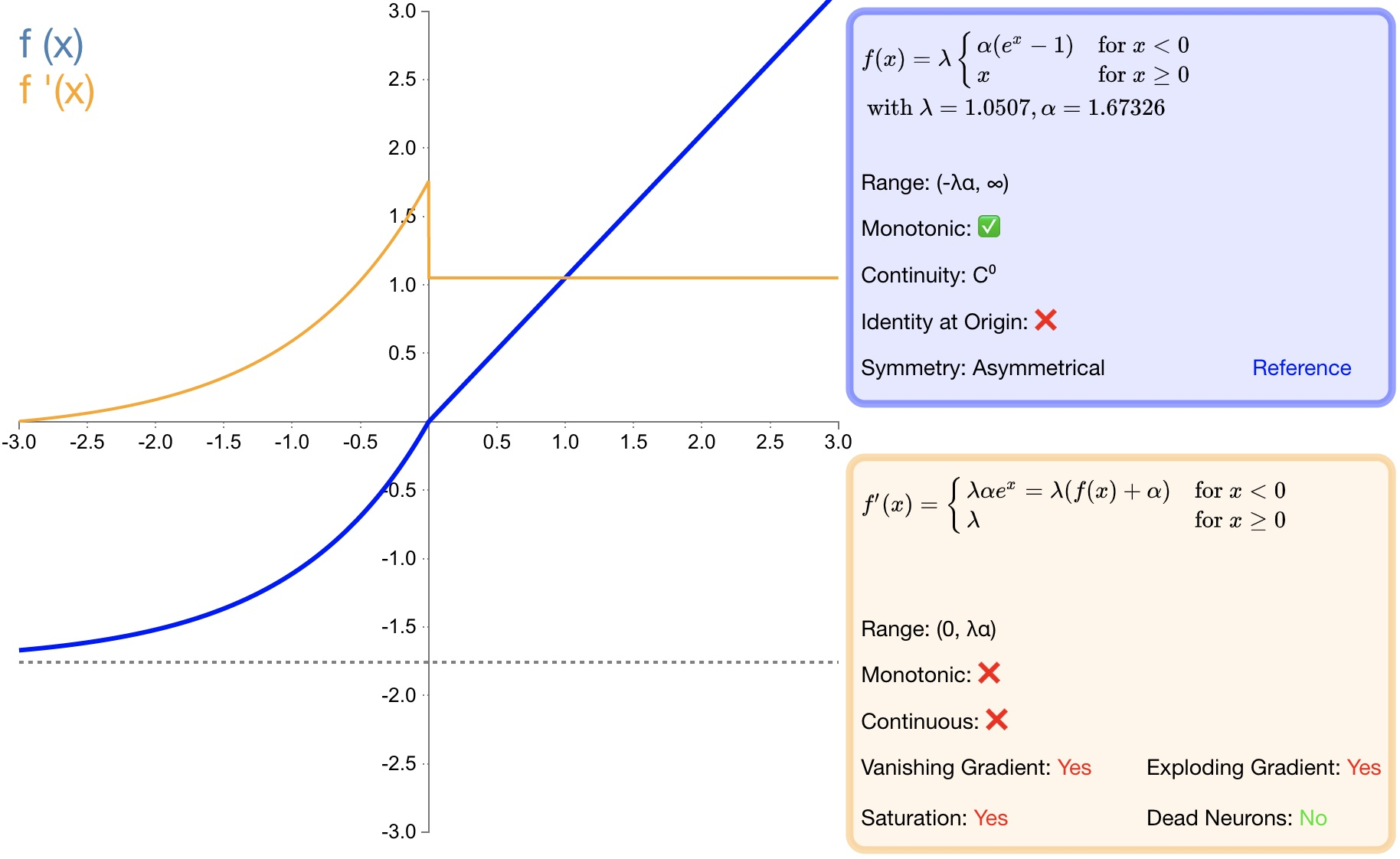- SELU 是 ELU 的一个变种。其中 λ 和 α 是固定数值（分别为 $1.0507$ 和 $1.6726$）;

 - 经过该激活函数后使得样本分布自动归一化到 $0$ 均值和单位方差;

 - 不会出现梯度消失或爆炸问题;

### 3.11. softsign

$$f(x)=\frac { x }{ \left| x \right| +1 }$$

$${ f }^{ ' }(x)=\frac { 1 }{ { (1+\left| x \right| ) }^{ 2 } }$$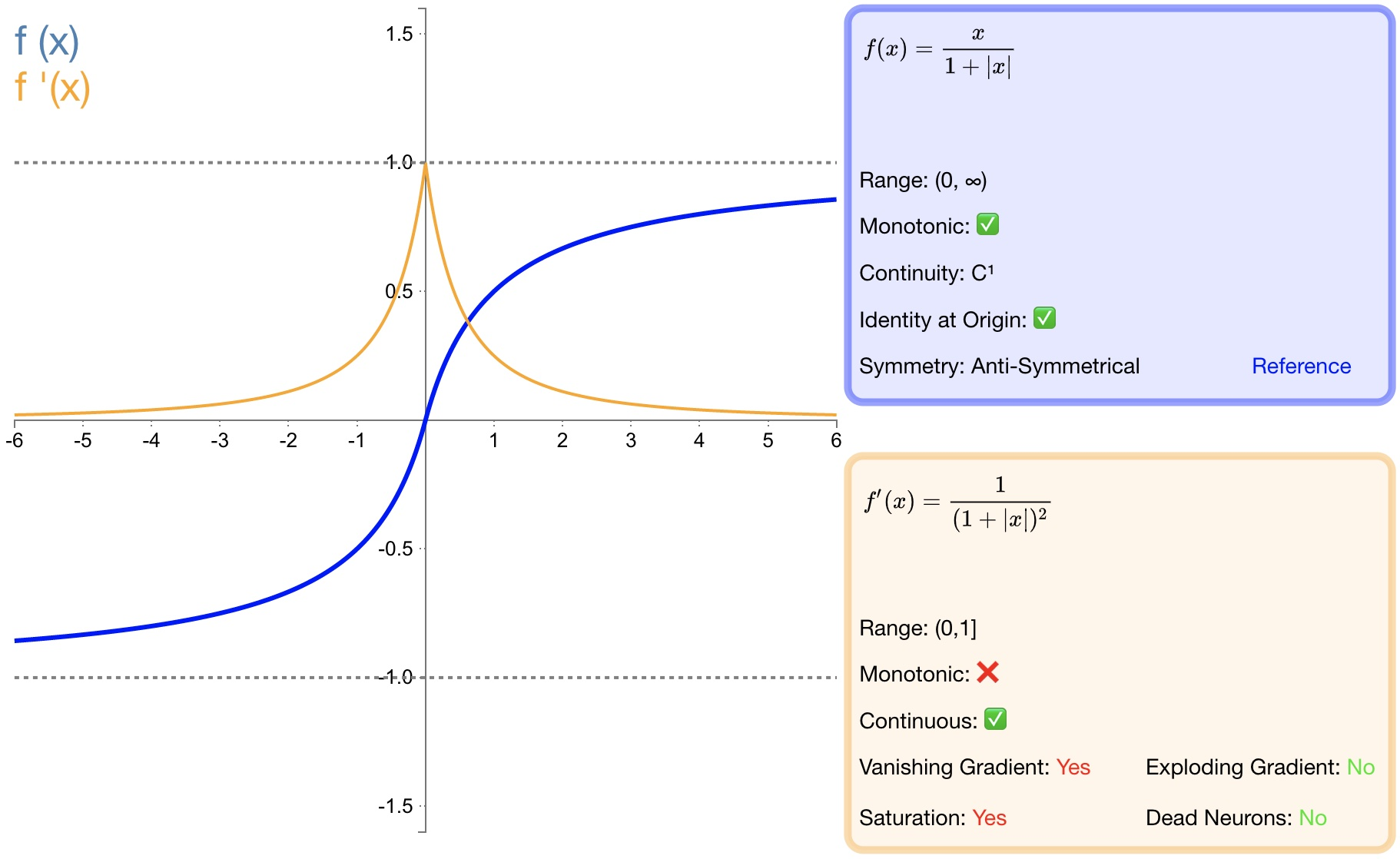- $softsign$ 是 $tanh$ 激活函数的另一个替代选择；

 - $softsign$ 是反对称、去中心、可微分，并返回 $-1$ 和 $1$ 之间的值；

 - $softsign$ 更平坦的曲线与更慢的下降导数表明它可以更高效地学习；

 - 导数的计算比$tanh$ 更麻烦；

### 3.12. softplus

$$f(x)=\ln { (1+{ e }^{ x }) }$$

$${ f }^{ ' }(x)=\frac { 1 }{ 1+{ e }^{ -x } }$$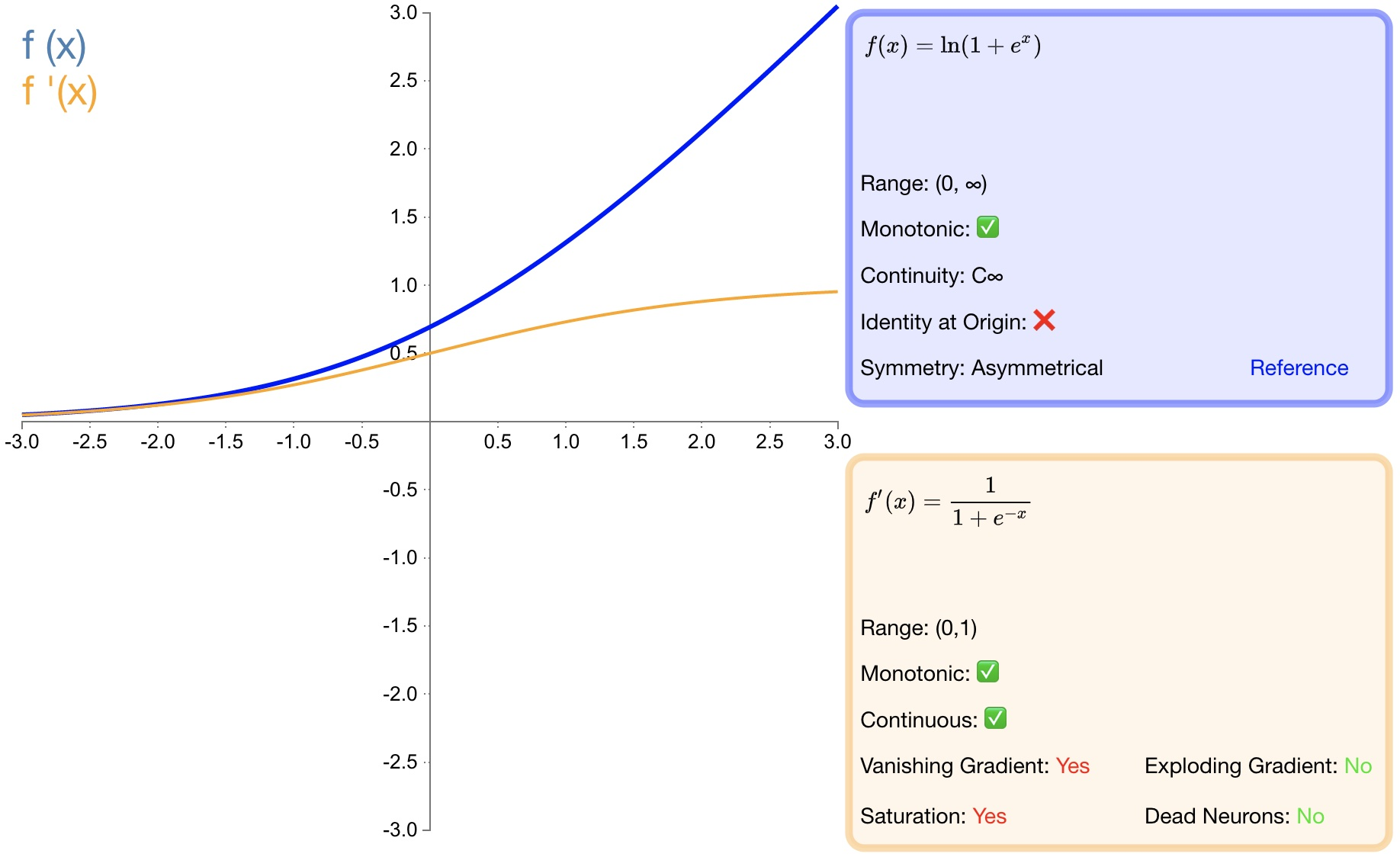- 作为 $relu$ 的一个不错的替代选择，$softplus$ 能够返回任何大于 $0$ 的值。

 - 与 $relu$ 不同，$softplus$ 的导数是连续的、非零的，无处不在，从而防止出现死神经元。

 - 导数常常小于 $1$ ，也可能出现梯度消失的问题。

 - $softplus$ 另一个不同于 $relu$ 的地方在于其不对称性，不以零为中心，可能会妨碍学习。

### 3.13. softmax

softmax 函数一般用于多分类问题中，它是对逻辑斯蒂（logistic）回归的一种推广，也被称为多项逻辑斯蒂回归模型(multi-nominal logistic mode)。假设要实现 k 个类别的分类任务，Softmax 函数将输入数据 $x_i$ 映射到第 $i$ 个类别的概率 $y_i$ 如下计算：

$$y_i=soft\max \left( x_i \right) =\frac{e^{x_i}}{\sum_{j=1}^k{e^{x_j}}}$$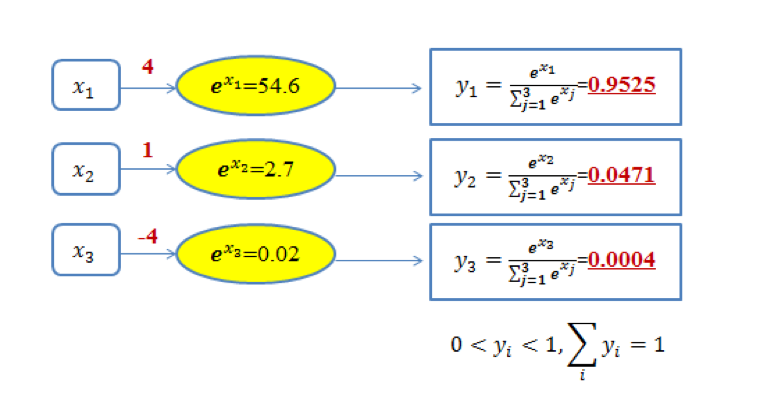### 3.14. swish

$$f\left( x \right) =x\cdot \sigma \left( x \right)$$

$swish$ 激活函数的图形如 图14 所示：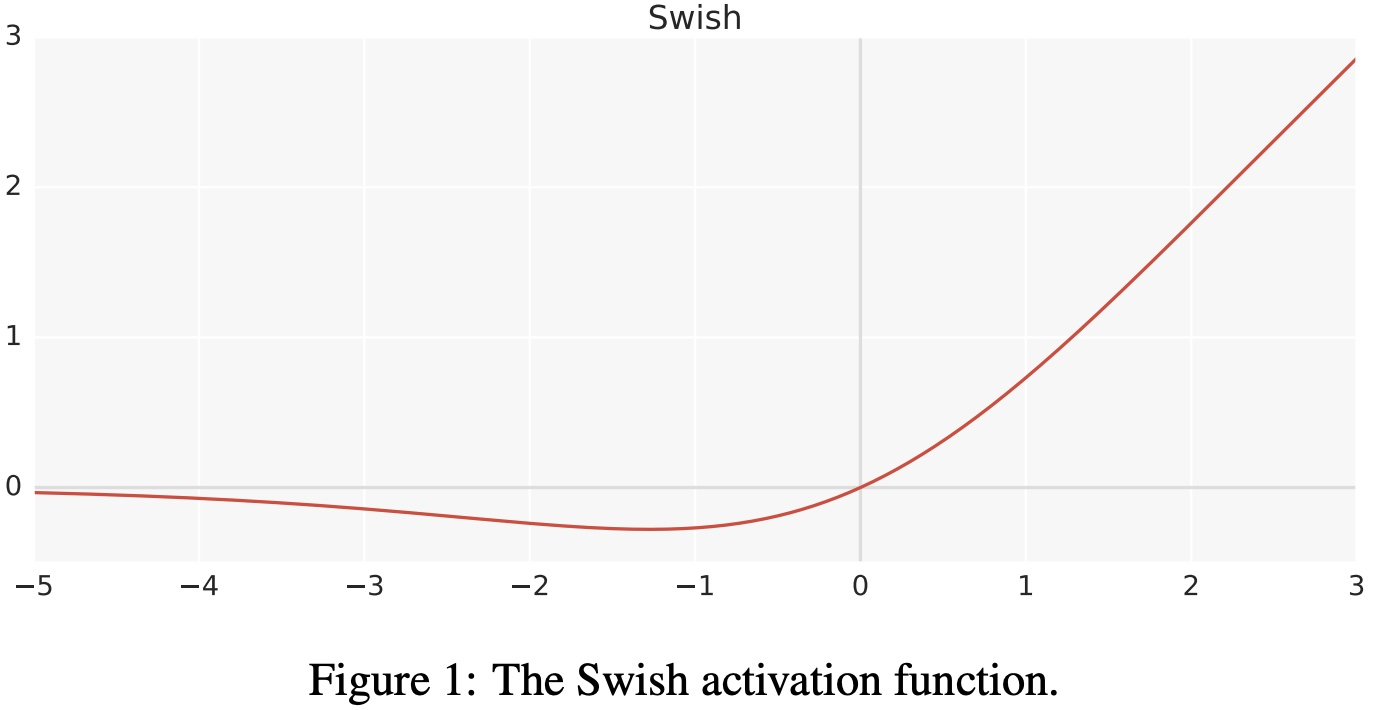$swish$ 激活函数的一阶导数如下：

$$f^{'}\left( x \right) =\sigma \left( x \right) +x\cdot \sigma \left( x \right) \left( 1-\sigma \left( x \right) \right)$$

$$f^{'}\left( x \right) = \sigma \left( x \right) +x\cdot \sigma \left( x \right) -x\cdot \sigma \left( x \right) ^2\\$$

$$f^{'}\left( x \right) = x\cdot \sigma \left( x \right) +\sigma \left( x \right) \left( 1-x\cdot \sigma \left( x \right) \right)$$

$$f^{'}\left( x \right) =f\left( x \right) +\sigma \left( x \right) \left( 1-f\left( x \right) \right)$$

$swish$ 激活函数的一阶和二阶导数的图形如 图15 所示：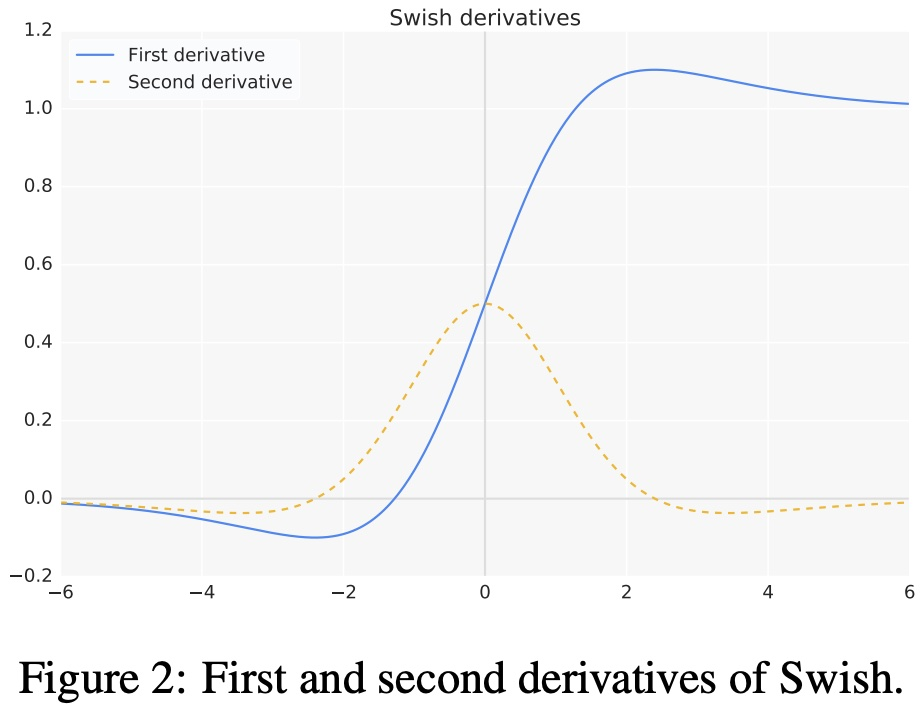$$f\left( x \right) =x\cdot \sigma \left( \beta x \right)$$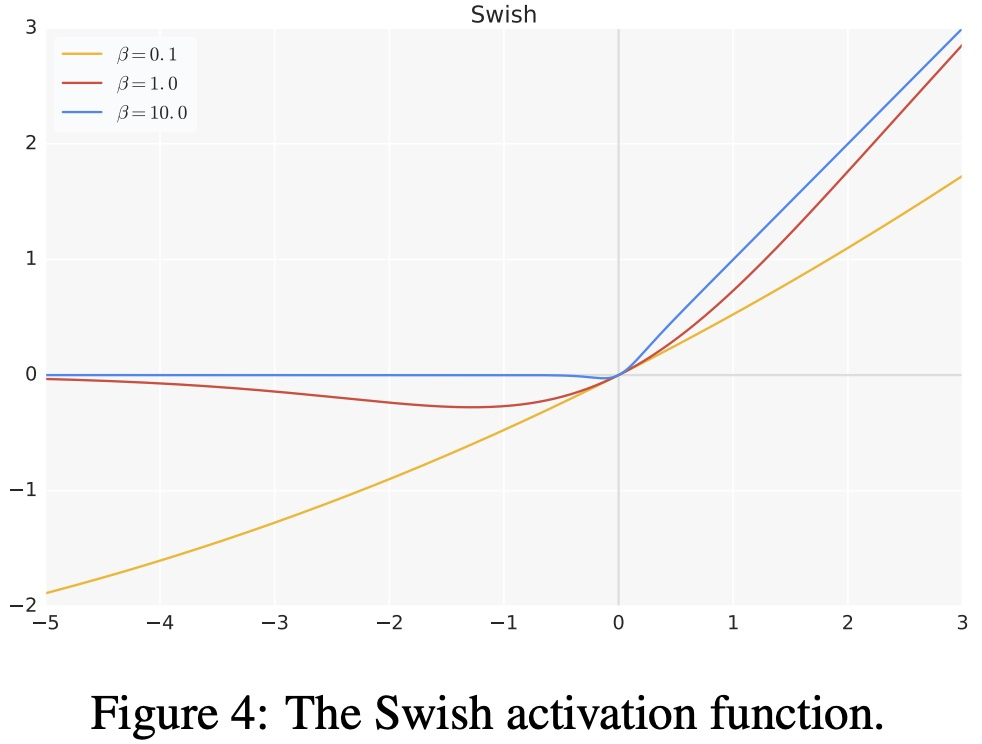- 当 $x>0$ 时，不存在梯度消失的情况；当 $x<0$ 时，神经元也不会像 ReLU 一样出现死亡的情况；

 - $swish$ 处处可导，连续光滑；

 - $swish$ 并非一个单调的函数；

 - 提升了模型的性能；

 - 计算量大；

### 3.15. hswish

$$f\left( x \right) =x\frac{\text{Re}LU6\left( x+3 \right)}{6}$$

$hard \ swish$ 和 $swish$ 激活函数对比如 图17 所示：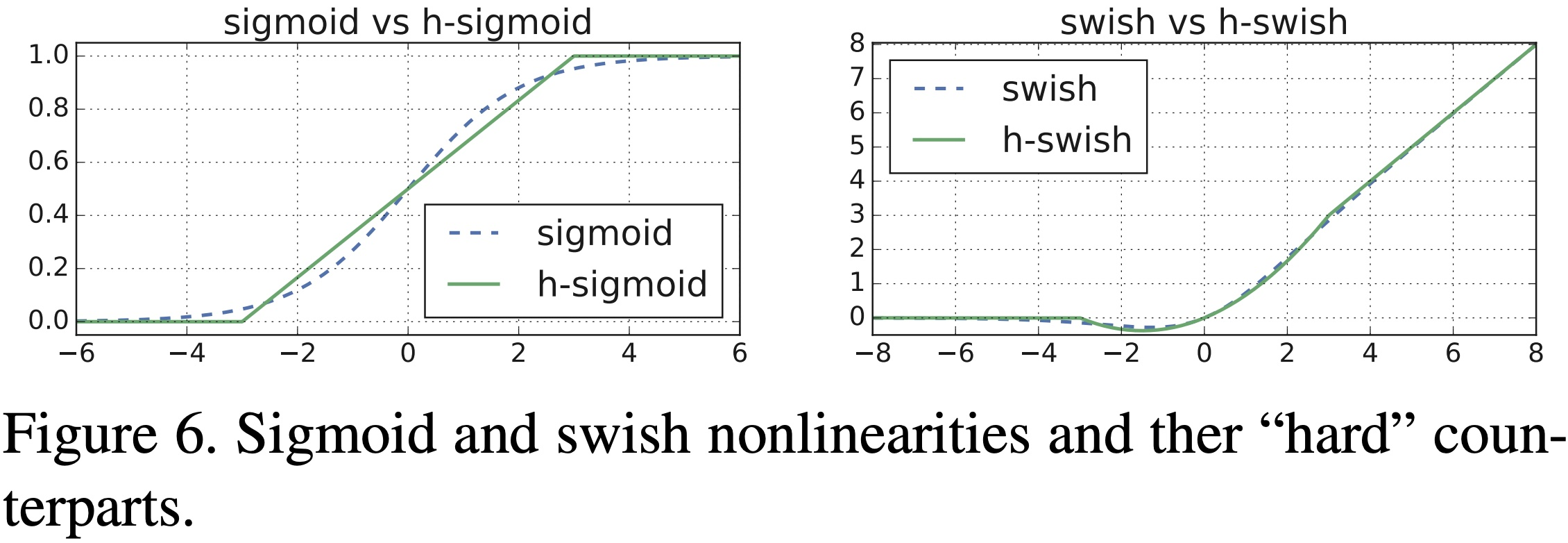- 与 $swish$ 相比 $hard \ swish$ 减少了计算量，具有和 $swish$ 同样的性质。

 - 与 $relu6$ 相比 $hard \ swish$ 的计算量仍然较大。

## 4. 激活函数的选择

 - 浅层网络在分类器时，$sigmoid$ 函数及其组合通常效果更好。

 - 由于梯度消失问题，有时要避免使用 $sigmoid$ 和 $tanh$ 函数。

 - $relu$ 函数是一个通用的激活函数，目前在大多数情况下使用。

 - 如果神经网络中出现死神经元，那么 $prelu$ 函数就是最好的选择。

 - $relu$ 函数只能在隐藏层中使用。

 - 通常，可以从 $relu$ 函数开始，如果 $relu$ 函数没有提供最优结果，再尝试其他激活函数。

## 5. 激活函数相关问题

### 5.2. 为什么 $tanh$ 的收敛速度比 $sigmoid$ 快？

$$\tan\text{h}^{'}\left( x \right) =1-\tan\text{h}\left( x \right) ^2\in \left( 0,1 \right)$$

$$s^{'}\left( x \right) =s\left( x \right) \left( 1-s\left( x \right) \right) \in \left( 0,\frac{1}{4} \right]$$

### 5.3. sigmoid 和 softmax 有什么区别？

 - 二分类问题时 $sigmoid$ 和 $softmax$ 是一样的，都是求 $cross \ entropy \ loss$ ，而 $softmax$ 可以用于多分类问题。

 - $softmax$ 是 $sigmoid$ 的扩展，因为，当类别数 $k=2$ 时，$softmax$ 回归退化为 $logistic$ 回归。

 - $softmax$ 建模使用的分布是多项式分布，而 $logistic$ 则基于伯努利分布。

 - 多个 $logistic$ 回归通过叠加也同样可以实现多分类的效果，但是 $softmax$ 回归进行的多分类，类与类之间是互斥的，即一个输入只能被归为一类；多 $logistic$ 回归进行多分类，输出的类别并不是互斥的，即"苹果"这个词语既属于"水果"类也属于"$3C$"类别。

Last modification：September 18th, 2021 at 10:27 am